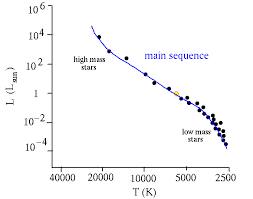## How to Calculate and Solve for Normally Occupied Positions | CeramicsThe image above represents normally occupied positions.

To compute for normally occupied positions, four essential parameters are needed and these parameters are Avogadro’s number (NA), Density (ρ), Atomic weight (AK) and Atomic weights (AG).

The formula for calculating normally occupied positions:

N = NAρ / (AK + AG)

N = Normally Occupied Positions
AK, AG = Atomic Weights
ρ = Density

Let’s solve an example;
Find the normally occupied positions when the avogadro’s number is 6.022e+23, atomic weight is 12, atomic weight is 16 and the density is 10.

This implies that;

NA = Avogadro’s Number = 6.022e+23
AK, AG = Atomic Weights = 12, 16
ρ = Density = 10

N = NAρ / (AK + AG)
N = (6.022e+23)(10)/(12 + 16)
N = (6.022e+24)/(28)
N = 2.15e+23

Therefore, the normally occupied positions is 2.15e+23.

Calculating the Density when the Normally Occupied Positions, the Avogadro’s Number, the Atomic Weights is Given.

ρ = N (AK + AG) / NA

Where;

ρ = Density
N = Normally Occupied Positions
AK, AG = Atomic Weights

Let’s solve an example;
Given that normally occupied positions is 20, the avogadro’s number is 6.022e+23, atomic weights is 18, 6.

This implies that;

N = Normally Occupied Positions =20
NA = Avogadro’s Number = 6.022e+23
AK, AG = Atomic Weights = 18, 6

ρ = N (AK + AG) / NA
ρ = 20 (18 + 6) / 6.022e+23
ρ = 20 (24) / 6.022e+23
ρ = 480 / 6.022e+23
ρ = 7.970e-22

Therefore, the density is 7.970e-22.